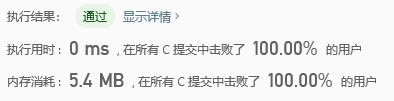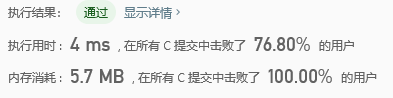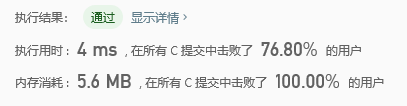# 数组简介 主要内容

• 数组
• 动态数组

## 数组简介

1. `数组`是一种基本的数据结构，用于按顺序`存储元素的集合。`但是元素可以随机存取，因为数组中的每个元素都可以通过数组`索引`来识别。
2. 数组可以有一个或多个维度。
3. `一维数组`也被称为线性数组
4. 数组 A 中有 6 个元素，则 A 的长度是 6 。

Initialize `int a0; int a1 = {1, 2, 3}` ;
Get length `int size = sizeof(a1) / sizeof(*a1);`
Access Element `a1`
Iterate all Elements* `for(int i=0; i<size; i++){...};`
Modify Element** `a1 = 4;`
Sort C++中有`sort(a1, a1 + size);` C中只有`qsort();`

*：遍历所有元素
** ：修改元素

# 程序练习

## 1. 寻找数组的中心索引

### 题目

• 给定一个整数类型的数组 nums，请编写一个能够返回数组“中心索引”的方法。
• 我们是这样定义数组中心索引的：数组中心索引的左侧所有元素相加的和等于右侧所有元素相加的和。
• 如果数组不存在中心索引，那么我们应该返回 -1。如果数组有多个中心索引，那么我们应该返回最靠近左边的那一个。

### 测试用例

nums = [1, 7, 3, 6, 5, 6]

### 代码实现

`函数judge`判断当前位置position是否满足

``````int judge(int* nums, int numsSize, int position){
int sum1=0, sum2=0;
for(int i=0; i<position; i++) sum1 += nums[i];
for(int j=position+1; j<numsSize; j++) sum2 += nums[j];
return sum1==sum2 ? 1:0;
}``````
``````int pivotIndex(int* nums, int numsSize){
for(int i=0; i<numsSize; i++)
if(judge(nums, numsSize, i)) return i;
return -1;
}``````

## 2. 至少是其他数字两倍的最大数

### 题目

• 在一个给定的数组nums中，总是存在一个最大元素 。
• 查找数组中的最大元素是否至少是数组中每个其他数字的两倍。
• 如果是，则返回最大元素的索引，否则返回-1。

### 代码实现

``````int judge(int* nums, int numsSize){
int position, max=0, i;
for(i=0; i<numsSize; i++){
if(max < nums[i]){
max = nums[i];
position = i;
}
}
for(int j=0; j<numsSize; j++){
if(j == position) continue;
if(max < 2*nums[j]) return 0;
}
return position+1;
}``````
``````int dominantIndex(int* nums, int numsSize){
return judge(nums, numsSize) ? (judge(nums, numsSize)-1):-1;
}``````【注】`judge函数``return position+1;`的原因：排除索引为0时产生的错误。

## 3. 加一

### 题目

• 给定一个由整数组成的非空数组所表示的非负整数，在该数的基础上加一。
• 最高位数字存放在数组的首位， 数组中每个元素只存储单个数字。
• 你可以假设除了整数 0 之外，这个整数不会以零开头。

### 代码

``````/**
* Note: The returned array must be malloced, assume caller calls free().
*/
int* plusOne(int* digits, int digitsSize, int* returnSize){
int* p;
*returnSize = digitsSize;
for(int i=digitsSize-1; i>-1; i--){
digits[i]++;
if(digits[i] > 9 && i) digits[i] = (digits[i])%10;
else if(digits[i]<10) return digits;
else;
}
if(*digits > 9){
(*returnSize) ++;
p =(int *)malloc(sizeof(int) *(*returnSize));
for(int i=digitsSize-1; i>=0; i--){
p[i+1] = digits[i]%10;
}
p = 1;
}
return p;
}``````### 优化

``````if(*digits > 9){
(*returnSize) ++;
p =(int *)malloc(sizeof(int) *(*returnSize));
for(int i=digitsSize-1; i>=0; i--){
p[i+1] = 0;
}
p = 1;
}``````